# 谷歌机器学习框架 ---- TensorFlowhttps://zhuanlan.zhihu.com/p/25547838

### 01 TensorFlow的编程模式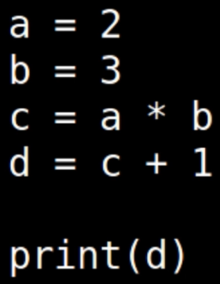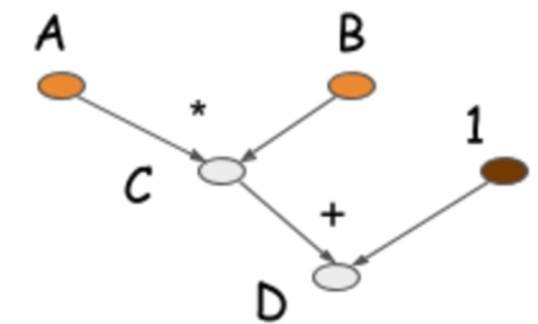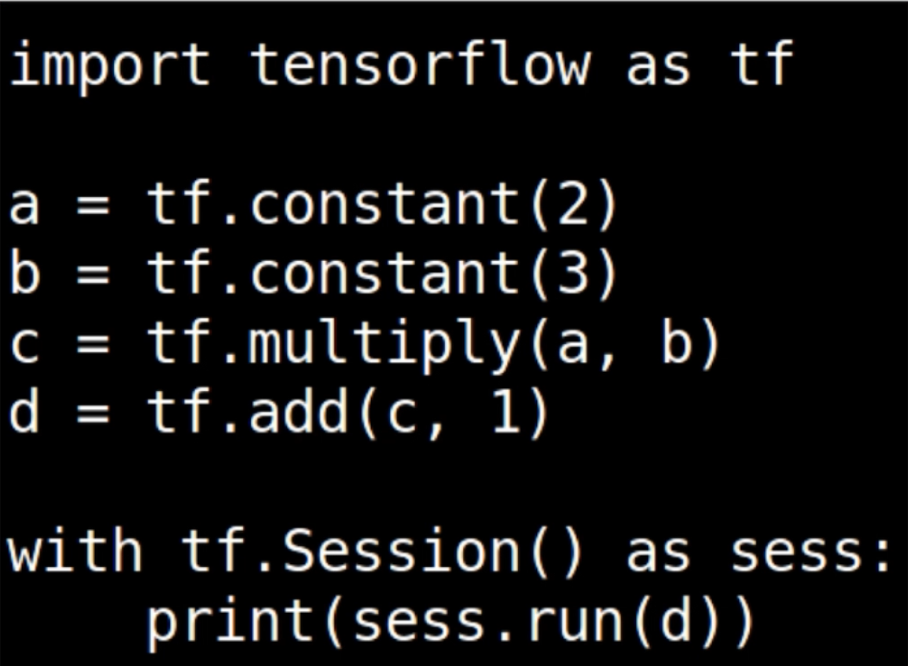### 02 TensorFlow的基础结构

TF的基础模型主要包括三个部分，即Tensor（张量），Graph（图），Session（会话）

Tensor与Tensor之间可能有各种数学运算，所有的Tensor运算结构就是一张Graph，而Session负责和设备交互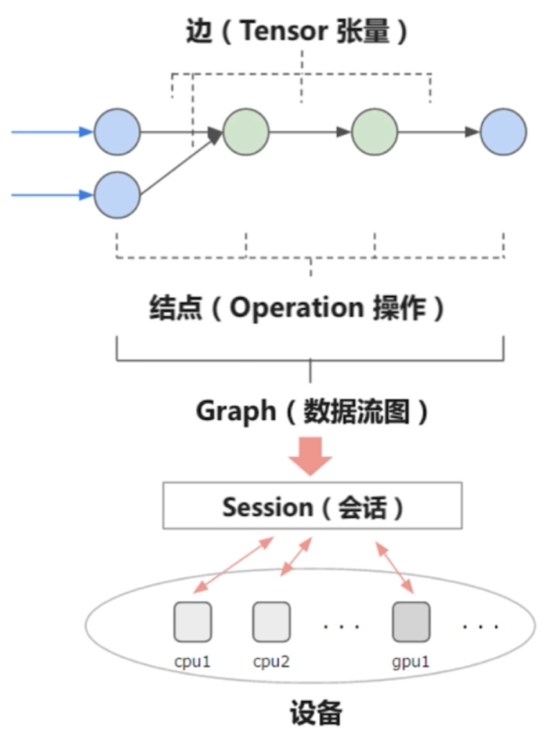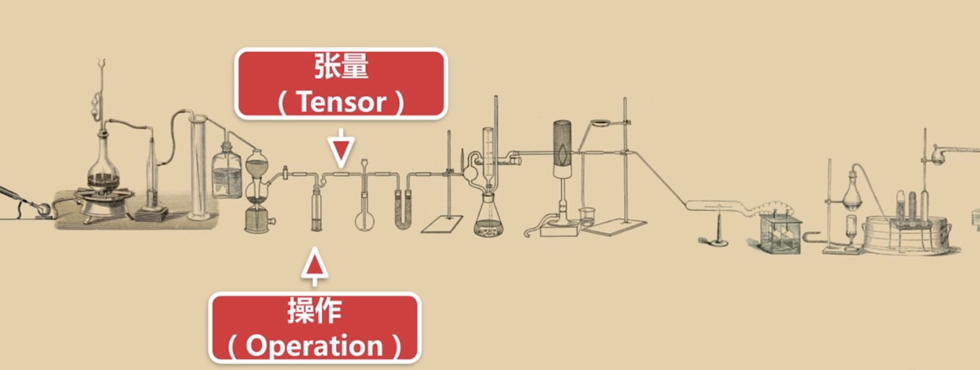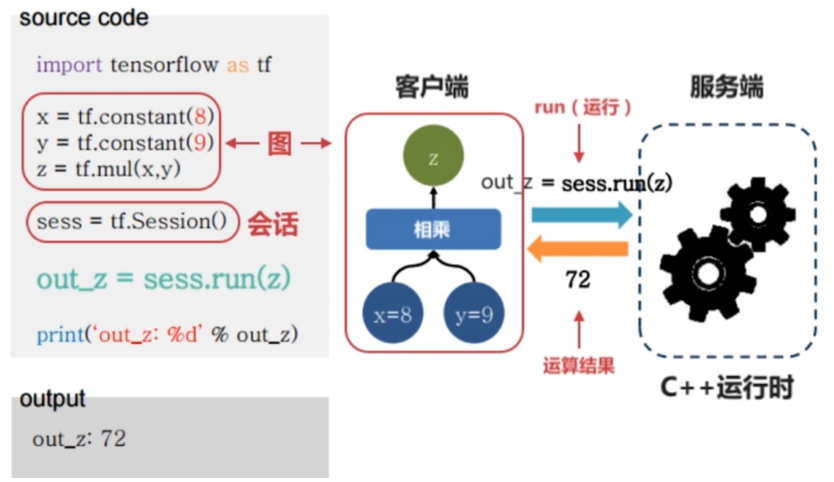### 03 Tensor(张量)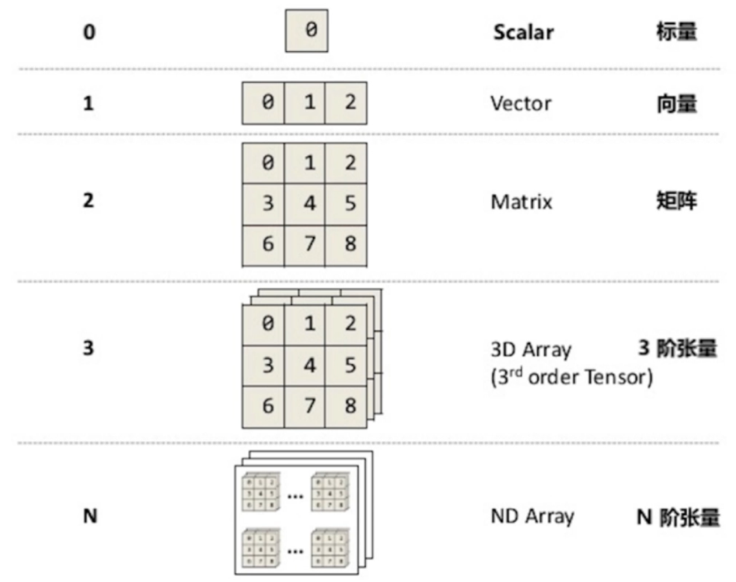Tensor有两个基本属性即：数据类型dtype和形状shape。分为Constant（常量）、Variable（变量）、Placeholder（占位符）、SparseTensor（稀疏张量）

SparseTensor的概念类似线性代数里的稀疏矩阵概念。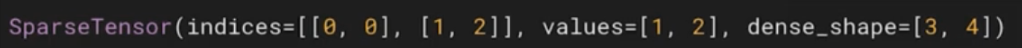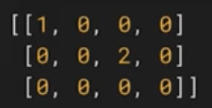indices定义value中数值的下标索引

Tensor表示法：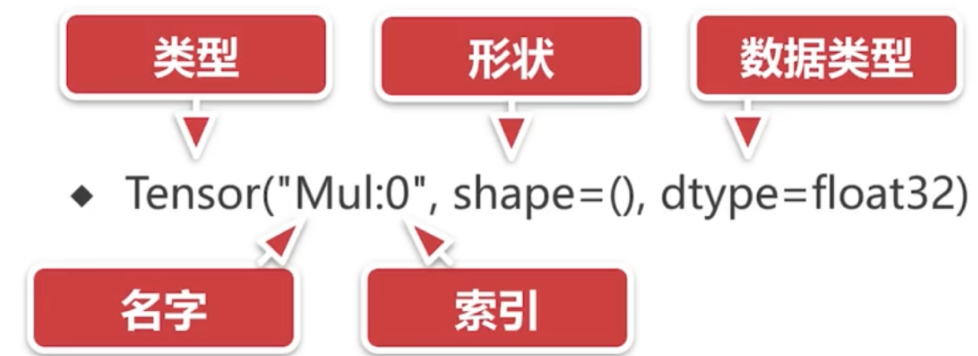基本编程模式：

``````import tensorflow as tf

# 创建一个常量 Operation(操作)
hw = tf.constant("Hello World! I love Tensorflow !")

# 启动一个Tensorflow的Session()
sess = tf.Session()

# 运行 Graph（计算图）
print(sess.run(hw))

# 关闭Session（会话）
sess.close()``````

### 04 可视化利器TensorBoard

1.用TensorFlow保存图的信息到日志中

``tf.summary.FileWriter("日志保存路径", sess.graph)``

2.用TensorFlow读取并展示日志

``tensorboard --logdir=日志所在路径``
``````import tensorflow as tf

# 构造图（Graph）的结构
# 用一个线性方程的例子 y = w * x + b
w = tf.Variable(2.0, dtype=tf.float32, name="weight")
b = tf.Variable(1.0, dtype=tf.float32, name="bias")
x = tf.placeholder(dtype=tf.float32, name="input")

with tf.name_scope("output"):   # 输出的命名空间
y = w * x + b

# 定义保存日志的路径
path = './log'

# 创建用于初始化所有变量的操作
init = tf.global_variables_initializer()

# 创建Session
with tf.Session() as sess:
sess.run(init)  # 初始化变量
writer = tf.summary.FileWriter(path, sess.graph)
result = sess.run(y, {x: 3.0})
print("y = %s" % result)``````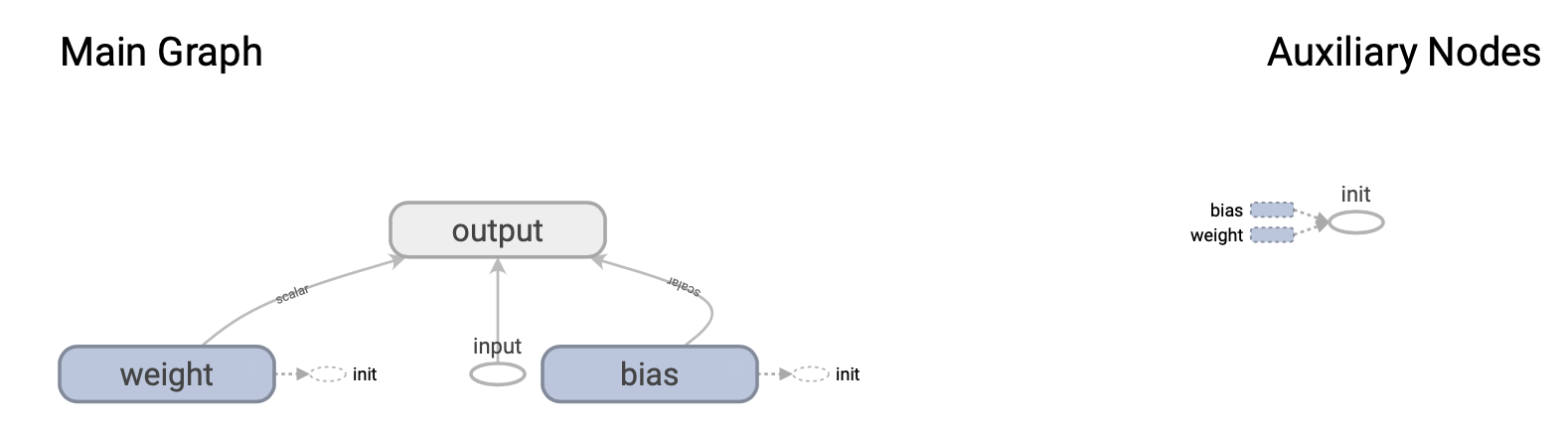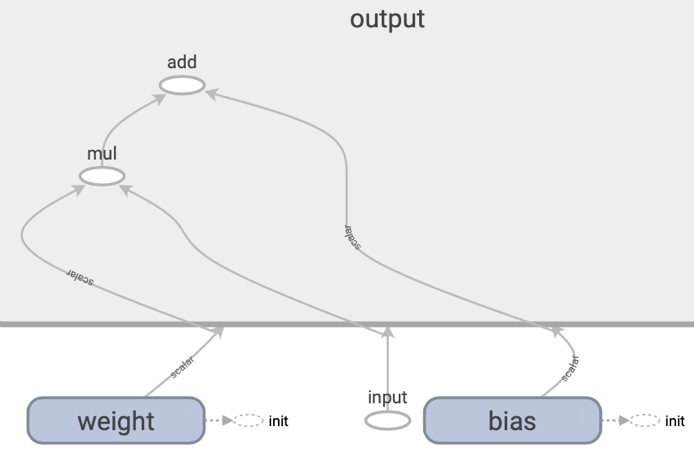-- 展开阅读全文 --

« 上一篇 07-24